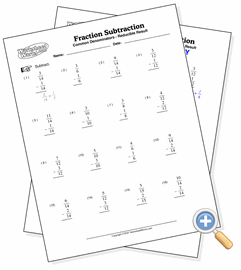# With Common Denominators

## Fraction SubtractionDevelop subtraction skills with simple fractions having common denominators

These problems are the first that students new to fraction subtraction will need to learn. Since the denominators are always the same, they only need to subtract the second numerator from the first. A pie chart or other physical media can be helpful here so that the student understands the meaning behind the fractions.